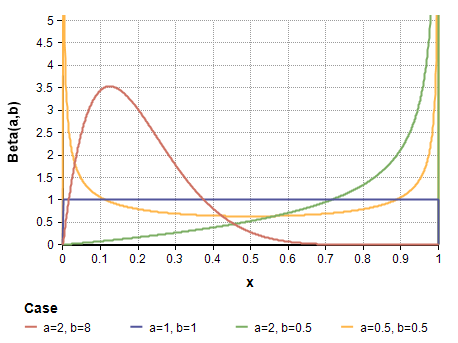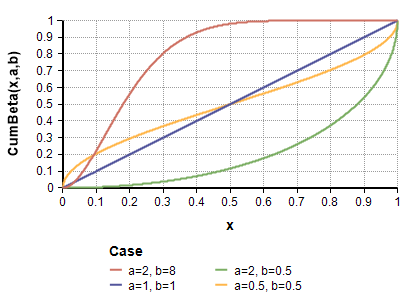# Beta distribution

(Redirected from Beta)

Release: 4.6  •  5.0  •  5.1  •  5.2  •  5.3  •  5.4  •  6.0  •  6.1  •  6.2  •  6.3  •  6.4

The beta distribution is a bounded continuous distribution. If is often used to express an uncertainty in a proportion, frequency, or percentage, which are all quantities between 0 and 1. With positive «x» and «y» parameters and arbitrary «lower» and «upper» bounds, it is also called a Pert distribution, and in this form is sometimes used as a smooth bell-shaped variation appropriate where a Triangular might otherwise be used. The beta distribution can take on several shapes including bell-shaped unimodal (when a,b>1), bimodal (when 0<a,b<1), uniform (when a=b=1), exponentially decaying (when 0<a<1<b) or exponentially increasing (when 0<b<1<a).

A Beta( n+1, m+1 ) is sometimes used as an estimate for the proportion of individuals with a given trait after observing n individuals with the trait and m individuals without the trait.## Functions

### Beta(a, b, lower, upper)

The Beta distribution.

Creates a continuous distribution of numbers between 0 and 1 with a/(a + b) representing the mean, if the optional parameters «lower» and «upper» are omitted. For bounds other than 0 and 1, specify the optional «lower» and «upper» bounds to offset and expand the distribution.

«a» and «b» must be positive.

The probability density of the beta distribution is given by

### DensBeta(x, a, b, lower, upper)

The probability density at «x», which for «lower»=0 and «upper»=1 is given by

$\displaystyle{ p(x) = {1\over{BetaFn(a, b)}} x^{a-1} (1-x)^{b-1} }$

where $\displaystyle{ 0 \le x \le 1 }$.

More generally, with $\displaystyle{ z = (x-lower)/(upper-lower) }$, the density is

$\displaystyle{ p(x) = {1\over{(upper-lower) BetaFn(a,b)}} z^{a-1} (1-z)^{b-1} }$

### CumBeta(x, a, b, lower, upper)

The cumulative density, i.e., the probability that the outcome is less than or equal to «x».The cumulative beta distribution is given by

$\displaystyle{ F(x) = BetaI( (x-lower) / (upper-lower), a,b) }$

where BetaI(x,a,b) is the regularized incomplete beta function (sometimes written as $\displaystyle{ I_x(a,b) }$).

### CumBetaInv(p, a, b)

The inverse cumulative density, also called the quantile function, which returns the value x with a «p» probability of being larger than the true value. Returns the value x for which there is a «p» probability that the outcome is less than or equal to x. This is the inverse of the CumBeta function.

## When to use

Use a beta distribution if the uncertain quantity is bounded by 0 and 1 (or 100%), is continuous, and has a single mode. This distribution is particularly useful for modeling an opinion about the fraction of a population that has some characteristic. For example, if you have observed n members of the population, of which r display the characteristic c, you can represent the uncertainty about the true fraction with c using a beta distribution with parameters «x» = r+1 and «y» = n - r + 1.

If the uncertain quantity has lower and upper bounds other than 0 and 1, include the «lower» and «upper» bounds parameters to obtain a transformed beta distribution. The transformed beta is a very flexible distribution for representing a wide variety of bounded quantities.

## Statistics

Theoretical statistics (i.e., in the absence of sampling error) for the beta distribution are as follows.

• Mean = a / (a+b)
• Mode = $\displaystyle{ { {a-1} \over {a+b-2} } }$, when $\displaystyle{ a,b\gt 1 }$
• Variance = $\displaystyle{ {{ab}\over{(a+b+1)(a+b)^2}} }$
• Skewness = $\displaystyle{ { {2(b-a)\sqrt{a+b+1} }\over{(a+b+2)\sqrt{ab}}} }$
• Kurtosis = $\displaystyle{ { {6 ( (a-b)^2 * (a+b+1) - a b (a+b+2) ) } \over { ( a b (a+b+2) (a+b+3))} } }$

## Parameter Estimation

Suppose D contains sampled historical data indexed by I, and you want to estimate the «X» and «Y» parameters of the beta distribution from this historical data. With your data in D normalized to be between the known bounds of 0 and 1, the parameters can be obtained from the following estimation formulas:

X := Local m := Mean(D, I);
Local v := Variance(D, I);
(m^2 - m^3 - v*m) / v
Y := Local m := Mean(D, I);
Local v := Variance(D, I);
(m*(1 - m)^2 - v * (1 - m)) / v

When the range is given and over something other than [0, 1], the above estimation formula apply with D replaced with (D - «lower»)/(«upper» - «lower»). Maximum likelihood estimation of all four parameters when «lower» and «upper» are not known is difficult, but worked out in Johnson, Kotz and Balakrishan (1994), Continuous Univariate Distributions, 2ne ed., Volume II, p. 221-235, John Wiley & sons.

A nice property of the Beta distribution is that it is a conjugate prior for a Bernoulli process -- i.e., a biased coin flip. The beta distribution denotes your current belief about the probability of success. If you start with a prior of Beta(a,b), and then observe a success, you simple add one to the first parameter to get the posterior. If you observe a success, you just add 1 to the second parameter to get the posterior.

• Prior: P(k) = Beta(a,b)
• Posterior: P(k|success) = Beta(a+1,b)
• Posterior: P(k|failure) = Beta(a,b+1)

This generalizes naturally to the observation of s successes and f failures:

Posterior: P(k | s successes, f failures) = Beta(a+s,b+f)